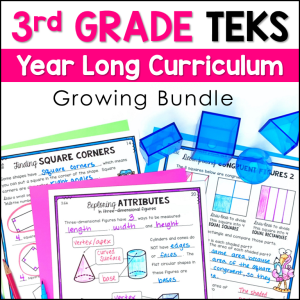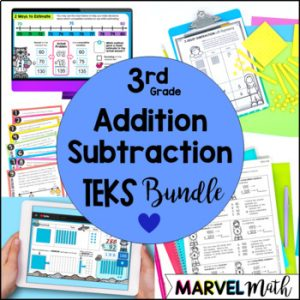Save 10% on your first order with code EXTRA10

# 3rd Grade Addition & Subtraction No Prep Games

\$5.00\$134.25\$26.25
SKU: 7115829 Category:

## Description

Your students will love this set of No Prep Addition, Subtraction, and Counting Coins Games. These FUN & ENGAGING games cover addition and subtraction with regrouping, estimation using rounding and compatible numbers, one and multi-step word problems, and counting a collection of coins.

⭐ There are student recording sheets for every game to add accountability at your math stations.

⭐ The color and blackline versions of each game are included!

⭐ There is a key for each game, to make the perfect self-checking math station.

❌ There are no pieces to cut out. Just print these games & laminate or place them in plastic sleeves.

What’s Included:

• 9 Games
• Color and Blackline versions of each game
• Keys for each game
• Student Recording Sheets for each game

Great ways to use these Math Games:

• Math Station
• Guide a Small Group Lesson
• Tutoring Sessions
• End of Unit Review
• STAAR Review

Topics include:

• Addition and Subtraction with Regrouping
• One-step word problems
• Multi-Step word problems
• Estimating Sums and Differences with rounding and compatible numbers
• Representing word problems with equations
• Finding the value of a collection of coins and bills

Included Games:

1.) Box It Up: 2-Digit Addition: Basic Practice

2.) Box It Up: 3-Digit Addition: Basic Practice

3.) Box It Up: 2-Digit Subtraction: Basic Practice

4.) Box It Up: 3-Digit Subtraction: Basic Practice

5.) Sums & Differences: 3-Digit Addition & Subtraction Practice emphasizing Vocabulary

6.) Shooting Hoops: Word Problem Practice

7.) S’more Word Problems: Representing Word Problems with Equations

8.) Popsicle Bump: Estimating Sums & Differences using Rounding and Compatible Numbers

9.) Collecting Coins: Adding collections of coins together to find the total value

TEKS Alignment:

3.4A: Solve with fluency one-step and two-step problems involving addition and subtraction within 1,000 using strategies based on place value, properties of operations, and the relationship between addition and subtraction.

3.5A: Represent one- and two-step problems involving addition and subtraction of whole numbers to 1,000 using pictorial models, number lines, and equations.

Supporting Standards

3.4B: Round to the nearest 10 or 100 or use compatible numbers to estimate solutions to addition and subtraction problems.

3.4C: Determine the value of a collection of coins and bills.